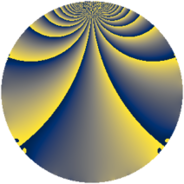# Properties

 Label 3549.2.bfLevel $3549$ Weight $2$ Character orbit 3549.bf Rep. character $\chi_{3549}(698,\cdot)$ Character field $\Q(\zeta_{6})$ Dimension $782$ Sturm bound $970$

# Related objects

## Defining parameters

 Level: $$N$$ $$=$$ $$3549 = 3 \cdot 7 \cdot 13^{2}$$ Weight: $$k$$ $$=$$ $$2$$ Character orbit: $$[\chi]$$ $$=$$ 3549.bf (of order $$6$$ and degree $$2$$) Character conductor: $$\operatorname{cond}(\chi)$$ $$=$$ $$273$$ Character field: $$\Q(\zeta_{6})$$ Sturm bound: $$970$$

## Dimensions

The following table gives the dimensions of various subspaces of $$M_{2}(3549, [\chi])$$.

Total New Old
Modular forms 1026 862 164
Cusp forms 914 782 132
Eisenstein series 112 80 32

## Trace form

 $$782q + 370q^{4} + 12q^{6} + 9q^{7} - 2q^{9} + O(q^{10})$$ $$782q + 370q^{4} + 12q^{6} + 9q^{7} - 2q^{9} + 9q^{15} - 332q^{16} - 2q^{18} - 7q^{21} - 10q^{22} - 309q^{25} + 48q^{28} - 20q^{30} + 39q^{31} - 16q^{36} - 11q^{37} - 162q^{40} + 74q^{42} + 12q^{43} + 3q^{45} + 90q^{48} - 7q^{49} + 16q^{51} + 27q^{54} - 6q^{55} + 26q^{57} + 60q^{58} - 55q^{60} + 59q^{63} - 444q^{64} - 141q^{66} + 66q^{67} + 33q^{69} - 20q^{70} + 34q^{72} - 60q^{73} + 6q^{75} + 12q^{76} - 61q^{79} + 30q^{81} - 12q^{84} + 2q^{85} - 111q^{87} - 92q^{88} - 13q^{93} - 30q^{96} - 33q^{97} - 22q^{99} + O(q^{100})$$

## Decomposition of $$S_{2}^{\mathrm{new}}(3549, [\chi])$$ into newform subspaces

The newforms in this space have not yet been added to the LMFDB.

## Decomposition of $$S_{2}^{\mathrm{old}}(3549, [\chi])$$ into lower level spaces

$$S_{2}^{\mathrm{old}}(3549, [\chi]) \cong$$ $$S_{2}^{\mathrm{new}}(273, [\chi])$$$$^{\oplus 2}$$